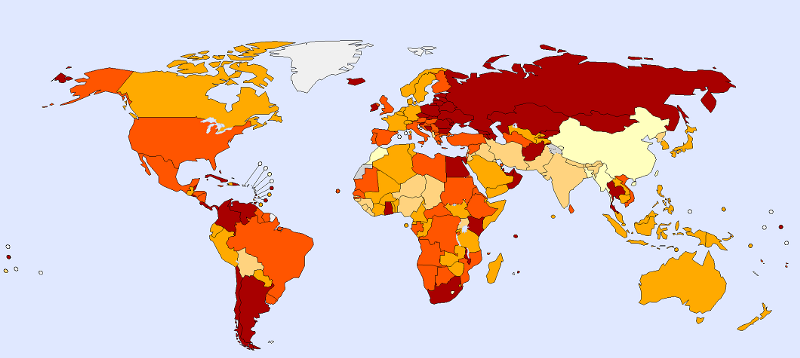﻿ Applied Roots

# Application of Hypothesis Testing and Spearman’s rank correlation coefficient to demystify Suicides worldwideTo show the use of statistical methods in real-world problems, for finding out if there is some statistical significance of an assumption we make after primary analysis of a data set.

Here we will explain and apply two well known statistical techniques:
1. Hypothesis Testing
2. Spearman’s rank correlation coefficient

The results of these statistical experiments will give us concrete proof of the assumptions we make after analyzing a set of data.